2022 Waec Objective Question And Answers – Free WAEC2022 Waec mathematics question and answers: Most likely Weac Mathematics answer 2022 to Objectives Essay question is available here. Candidates that applied for the West African Examination Council (WAEC) SSCE Examination will write their Waec mathematics paper on Monday 20th September 2021.

The Weac mathematics question and answer 2021 for preparation towards Waec start exam paper 2 & 1: Essay & Objective which will commence from 9:30 am – 12:00 pm and end by 3:00 pm – 4:30 pm.

In this post, we will be posting samples of the Waec mathematics questions for candidates that will participate in the examination.

Note that below is the questions from Waec mathematics past questions and answers that we feel are likely questions for preparation.

2022 Waec Mathematics Question and Answers ( Most likely Questions to Expect)

1. Which of these numbers is not less than -2?

1. -1,
2. -3,
3. -4,
4. -5.
5. -6

Solution Solving: -2 is greater than -2, -3, -4, -5, -6 and so on. But -1 is greater than -2.

The Correct Answer is A): Because it is greater than -2.

2. -6 is greater than -2 but less than -7? True or false

1. True
2. Both
3. False
4. None of the above

Solving for the solution: -6 is greater than any number from -7 to the negative infinity but less than any number from -5 to the positive infinity.

The Correct Answer is C): Because -6 is not greater than -2 and it is not less than -7.

3. Add 26b + 12a + 16a – 4b

1. 22b + 28a
2. -22b + 28a
3. 22b – 28a
4. -22b – 28a

Solution Solving for Questions 3: Firstly, you have to Collect like terms. Therefore, we have;

• 26b – 4b + 12a + 16a.
• Next, we have 26b – 4b = 22b
• And 12a + 16a = 28a
• Therefore, we have 22b + 28a

The Correct Answer is A): 22b + 28a

4. Multiply this equation: (x – 6)(2x + 7)?

1. 2×2 + 5x – 42
2. 2×2 – 5x – 42
3. 2×2 – 5x + 42
4. 2×2 + 5x + 42
5. -2×2 + 5x – 42Solving for question 4 solution: Here, we start by removing the bracket

Thus; 2×2 + 7x – 12x – 42

Hence, 2×2 – 5x – 42

The Correct Answer is B): 2×2 – 5x – 42

5. Factorise the following: 5×2 – 15x – 20?

1. 5(x+4)(x+1).
2. -2(x-4)(x+5)
3. -5(x+4)(x-1)
4. 5(x-4)(x+1)

Solution Solving to question 5: Firs find the L.C.M of 5, 15 and 20 = 5(x2 – 3x – 4).

• = 5(x2 – 4x + x – 4).
• = 5{x(x – 4) +1(x – 4)}.
• = 5(x-4)(x+1).

The Correct Answer is D): 5(x-4)(x+1)

6. In a school, 180 students offer mathematics or physics or both. If 125  offer mathematics and 105 offer physics. How many students offer mathematics only?
A.75       B. 80       C. 55       D. 125.
7. Find the value of x for which 3(24x + 3) = 96
A. 2       B. -2       C. ½       D. -1/2
8. The cost of renovating a 5m square room is N500. What is the cost of renovating a 10m square room?
A. N1,000       B. N2,500       C. 2,000       D. N10,000
9. Find the rate of change of the total surface area S of a sphere with respect to its radius r when r = 2
A. 8       B. 16       C. 10       D. 14
10. Differentiate (cosӨ + sinӨ)2 with respect to Ө.
A. 2cos2Ө       B. 2sin2Ө       C. -2cos2Ө       D. -2sin2Ө
11. A binary operation * on the set of rational numbers is defined as x * y = 2x + [x3 – y3/x + y]. find -1*2
A. 11       B. -11       C. 8       D. -8
12. A polynomial in x whose zeroes are 2, 1 and -3 is ______
A. x3 – 7x + 6
B. x3 + 7x – 6
C. x3 – 7x –  6
D. x3 + 7x + 6
13. Find the range of values of x for which 7x –  3 > 3x + 4
A. x < 7/4
B. x > 7/4
C. 7x < 4
D. -4x < 7
14. Some red balls were put in a basket containing 12 white balls and 16 blue balls. If the probability of picking a red ball from the basket is 3/7, how many red balls were introduced?
A. 13       B.20       C. 12       D. 21
15. Convert 12314 to a number in base 6.
A. 1056
B. 3016
C. 1036
D. 5016
16. Find the slope of the curve y = 3×3 + 5×2 – 3 at (-1, 5).
A. 1       B. -1       C. 19       D. -19
17. Find the area of the region bounded by y = x2 + x – 2 and x-axis
A. 9/2         B. -39/6         C. 8/3          D. 16/3
18. The minimum value of y = x2 – 4x – 5 is ______
A. 2          B. -2         C.13          D. -13

Waec General Mathematics Theory and Answer Paper 2, WASSCE

1. Copy and complete the table of values for the equation y = 2×2 – 7x – 9 for -3 ≤ x ≤ 6.
 X -3 – 2 -1 1 2 3 4 5 6 Y 13 -9 -14 -12 6
1. Using scales of 2 cm to 1 unit on the x-axis and 2 cm to 4 units on the y – axis, draw the graph of y = 2×2 – 7x – 9 for -3 ≤ x ≤ 6.
2. Use the graph to estimate the:
3. roots of the equation  2×2 – 7x = 26;

(ii)       coordinates of the minimum point of y;
(iii)       range of values for which  2×2 – 7x < 9.

A solution to Question 1

The Chief Examiner reported that the majority of the Candidates’ who attempted this question got right the complete table of values for which y = 2×2 – 7x – 9. However, many failed to plot the points on the graph correctly on the graph thereby losing marks. Also, they could not read up values from the graph to answer subsequent questions.
In part (a), the Candidates were expected to complete the table as follows:

 X -3 – 2 -1 1 2 3 4 5 6 Y 30 13 -9 -14 -15 -12 -5 6 212021 Waec mathematics question and answers

In part (b), Candidates would then use this table and the scale provided to draw the graph of the relation y = 2×2 – 7x – 9 and use this graph to answer the questions that followed.

In part (c), from the graph, the roots of the equation 2×2 – 7x = 26 were obtained by drawing a line through y = 17 to meet the curve y = 2×2 – 7x – 9. The required roots are – 2.2 ± 0.1 and 5.8 ± 0.1. The coordinates of the minimum point of y is 1.8 ± 0.1, -15 ± 0.4.                The ranges -1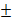0.1<x<4.5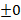Question 2

 Marks 1 2 3 4 5 Number of students m + 2 m – 1 2m – 3 m + 5 3m – 4

The table shows the distribution of marks scored by some students in a test.

1. If the mean mark is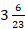, find the value of m.
2. Find the:

(i) inter-quartile range;
(ii) probability of selecting a student who scored at least 4 marks in the test.

The solution to Question 2

 x 1 2 3 4 5 Total f m+2 m-1 2m-3 m+5 3m-4 8m-1 fx m+2 2m-2 6m-9 4m+20 15m-20 28m-9

From the table,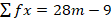and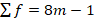, so that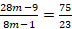and solving yields m = 3.
In part (b) (i), since m has been gotten to be 3, the table then becomes:

 x 1 2 3 4 5 f 5 2 3 8 5 Cf 5 7 10 18 23Therefore, interquartile range = 4 – 2 = 2.
Finally, in part (b) (ii), the probability of selecting a student who scored at least 4 marks is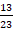whereas most Candidates’ made use of at most 4 marks to obtain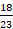NOTE THAT:

All the questions drop down are not exactly 2021 WAEC Mathematics questions and answers but likely WAEC Mathematics repeated questions and answers, so if you can practice the questions it can also be of help.